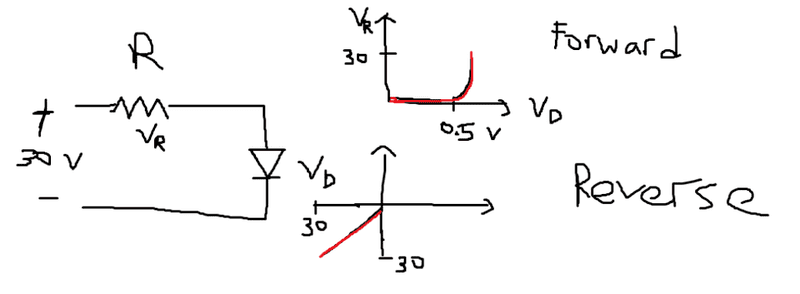# Exploring P-N Diode Voltage Drops

• quietrain
In summary, the machine extrapolated the data when the voltage across the resistor and diode reached 30V.

## Homework Statement

ok i was doing an experiment on p-n diodes
i was asked to plot graph of voltage across resistor VS voltage of diode
with a DC source varying from 0 to 30V
resistor 1k ohm## The Attempt at a Solution

i understand the forward bias where the graph shows that the voltage across the diode is very small at 0.5V max even when the voltage across the resistor is increasing like mad. it shows that most of the voltage drop occurs over the resistor and not the diode which is right

but the problem is the 2nd part. on the reverse bias.

why is the potential drop equal on both the resistor and the diode? namely 30 V each. since it is series connection, how can the total potential drop of 60V be more than my source's max 30V ?

have i reached breakdown voltage yet? because i read that avalanche braekdown usually occurs at >50V?
so my graph is still just reverse bias only?

thanks!

Exactly what connections did you make with the meter to measure the resistor voltage and diode voltage?

it was a x-y graphing machine plotter or sorts

so it plots the voltage of its x-y terminals

so i connected the x voltage measuring wires across the diode and the y across the resistor.

could it be the machine extrapolated itself? but i don't think that's the case, because it plots as i increase the source from 0 to 30V, so when source reaches 30V , the graph i obtain is as such :O

quietrain said:
it was a x-y graphing machine plotter or sorts

so it plots the voltage of its x-y terminals

so i connected the x voltage measuring wires across the diode and the y across the resistor.

could it be the machine extrapolated itself? but i don't think that's the case, because it plots as i increase the source from 0 to 30V, so when source reaches 30V , the graph i obtain is as such :O

So, the machine has two pairs of wires, one pair for measuring "x" and another pair for measuring "y"?

It sounds like there may have been a mix-up in your sensor wiring for the reverse bias testing. Perhaps the "ground" lead for both x and y were at the same location: the cathode of the diode.

gneill said:
So, the machine has two pairs of wires, one pair for measuring "x" and another pair for measuring "y"?

It sounds like there may have been a mix-up in your sensor wiring for the reverse bias testing. Perhaps the "ground" lead for both x and y were at the same location: the cathode of the diode.

oh i realized maybe i screwed up the units :(

will it make more sense if the voltage across the Y (resistor) become 0.03V ? that means the scale for Y is 3mV/cm and for X is 3V/cm

Yes, that would be better, but still not great. I would expect the reverse current to be on the order of a few microamps for a typical rectifier diode. Small signal diodes would do much better (lower) than that.

If you're getting 0.03V across the 1k resistor, then that's about 30 micro amps, which is high, but not totally impossible.

thank you!